#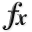N-D Test Functions E¶

class go_benchmark.Easom(dimensions=2)

Easom test objective function.

This class defines the Easom global optimization problem. This is a multimodal minimization problem defined as follows: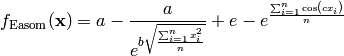Where, in this exercise,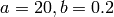and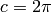.

Here,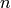represents the number of dimensions and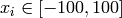for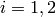.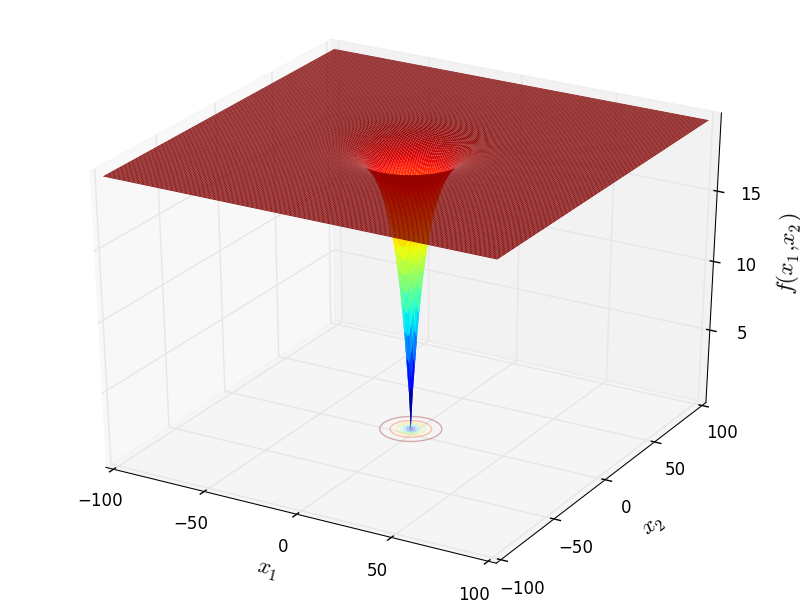Two-dimensional Easom function

Global optimum: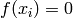for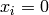forclass go_benchmark.EggCrate(dimensions=2)

Egg Crate test objective function.

This class defines the Egg Crate global optimization problem. This is a multimodal minimization problem defined as follows:Here,represents the number of dimensions andfor.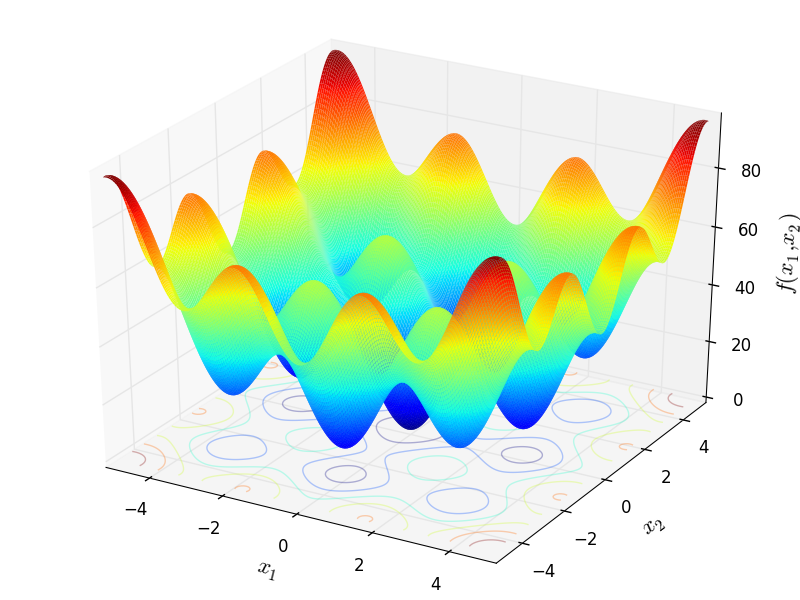Two-dimensional Egg Crate function

Global optimum:forforclass go_benchmark.EggHolder(dimensions=2)

Egg Holder test objective function.

This class defines the Egg Holder global optimization problem. This is a multimodal minimization problem defined as follows: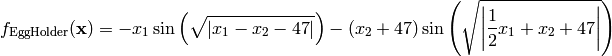Here,represents the number of dimensions and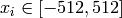for.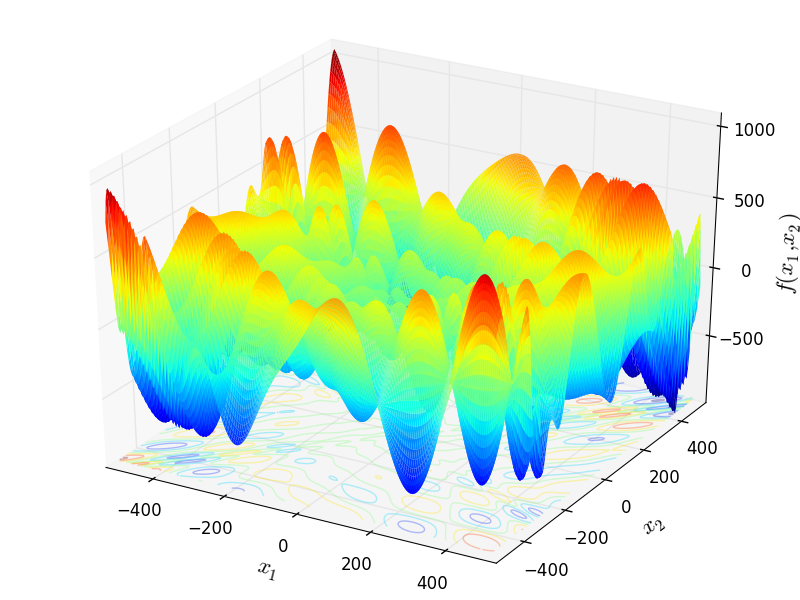Two-dimensional Egg Holder function

Global optimum: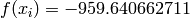for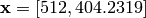class go_benchmark.ElAttarVidyasagarDutta(dimensions=2)

El-Attar-Vidyasagar-Dutta test objective function.

This class defines the El-Attar-Vidyasagar-Dutta function global optimization problem. This is a multimodal minimization problem defined as follows:Here,represents the number of dimensions andfor.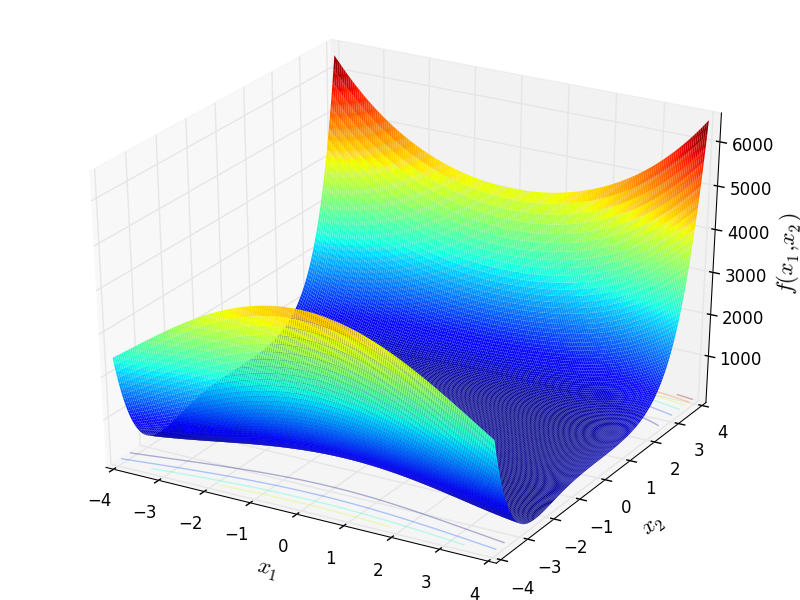Two-dimensional El-Attar-Vidyasagar-Dutta function

Global optimum:for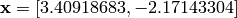class go_benchmark.Exp2(dimensions=2)

Exp2 test objective function.

This class defines the Exp2 global optimization problem. This is a multimodal minimization problem defined as follows:Here,represents the number of dimensions and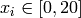for.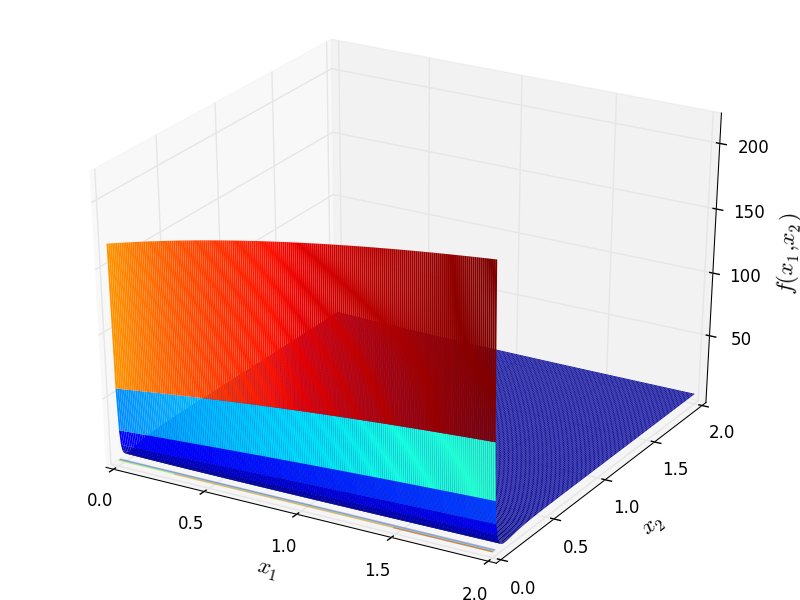Two-dimensional Exp2 function

Global optimum:for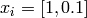class go_benchmark.Exponential(dimensions=2)

Exponential test objective function.

This class defines the Exponential global optimization problem. This is a multimodal minimization problem defined as follows: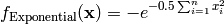Here,represents the number of dimensions and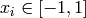for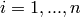.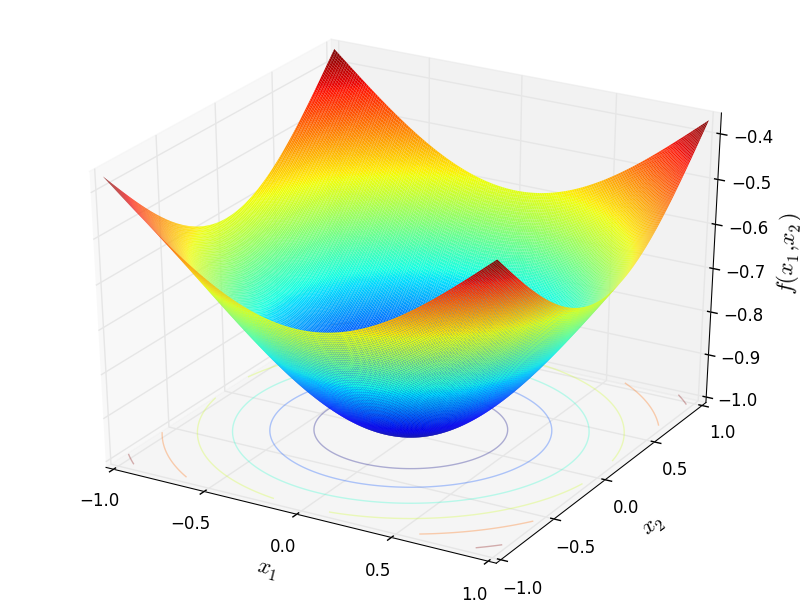Two-dimensional Exponential function

Global optimum:forfor#### Previous topic

N-D Test Functions D

#### Next topic

N-D Test Functions F